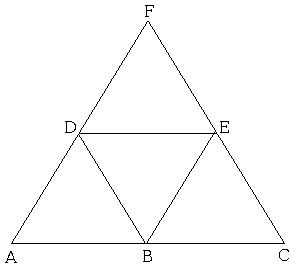# Two Colors - Three Points

Points in the plane are colored in two colors. Prove that it is always possible to find a monochromatic equilateral triangle, i.e., three points of the same color with all pairwise distances equal.

SolutionPoints in the plane are colored in two colors. Prove that it is always possible to find a monochromatic equilateral triangle, i.e., three points of the same color with all pairwise distances equal.

For convenience, name the colors red and blue.

Start with finding three collinear points A, B, C of the same color. Add points D, E, F as shown:There are 1 big and 4 small equilateral triangles. Assume A, B, C are red. If one of D or E is red, we are done. Otherwise, they both are blue. Then if F is red, triangle ACF is monochromatic. Otherwise, if F is blue, triangle DEF is monochromatic.

The same argument works for triangles of any shape, since the midlines of a triangle subdivide it into four smaller triangles of the same shape.

In passing, the claim above admits a generalization: there are monochromatic triangles of any shape. Furthermore, the above proof never used the fact that the given triangle is equilateral. We may remark that that condition was a red herring.

### References

1. R. B. J. T. Allenby, A. Slomson, How to Count: An Introduction to Combinatorics, CRC Press, 2011 (2nd edition)